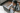# Knowing How To Find the Area and Perimeter of a Square

POSTED ON MARCH 29, 2023If you’re learning about the square, its area, and perimeter, you’ve come to the right place! The square is a special type of quadrilateral because all of its sides are equal. Let’s use this to our advantage when working with the square’s perimeter and area.

When you’re ready, head over to the next section and learn about the square’s area and perimeter with us!

## What Is the Area of a Square?

The area of the square is equal to the square of the length of its side and is measured in square units. Before we start solving using the formula for the area of the square, let’s understand what a square’s area represents. Let’s begin by remembering what we know about squares!

Juni Learning offers high-quality resources for geometry and other math topics through new math techniques. Let Juni Learning’s content allow you to master the Common Core standards. Just like those math concepts, Juni Learning’s catalog allows you to discover your identity as a learner.Take a look at these three different squares. Notice something special about them? A square is a special type of quadrilateral. The video you’ve just watched shows us what makes a square unique: the sides of a square have equal lengths. This means that if a square is broken down into smaller square units, the rows and columns will have the same number of squares.The square shown above is made up of 16 smaller squares (or tiles). Let’s say that each tile has side lengths of 1 unit. The square on the right shows you how these 16 tiles make up the larger one. If the area of one tile is 1 unit², the larger square has an area of 16 x 1 unit² = 16 unit². Meaning, the area of the square tells the number of square units needed to fill the given square.

#### Example 1

Apply what you’ve just learned to find the area of the square made up of 1 x 1 tiles as shown below.By taking a look at the square shown above, you can see that it will take 25 tiles each with an area of 1 cm² each. Hence, it takes 25 x 1 cm² = 25 cm² to fill the entire space of the square.This tells you that the area of the square shown above is 25 cm. There are instances, however, that we are only given the square’s side length. There is a way to find the area given the sides of a square.

Boost math confidence to the next level
Juni’s vetted instructors study at top US Universities and provide our students with the support and mentorship to grow their math skills.

## What Is the Formula for the Area of the Square?

Calculate the area of the square by squaring the length of its side. Since all sides of a square are equal in length, one side length is enough. When a square has a side length of s units, its area is equal to s x s = s² units.This means that all you need is the length of one side of the square and you’re good to go!

#### Example 2

To better understand the formula we’ve just shown you, let’s find the area of the square shown below.Taking a look at the square’s side, each side has a length of 12 inches. Using the formula for the square’s area, Area = s x s = s², multiply the side length by itself or simply square the side length.Hence, the square has an area of 12 x 12= 144 in².

#### Example 3

Remember the first three squares from this article? Use what you know about squares and their areas to calculate each of the three squares' areas.For each square, simply multiply the side lengths by itself or square the side lengths to find each of their areas. Here’s a table that summarizes the calculation for you.We’ve now covered all the bases for the square’s area, so it’s time to refresh what we know of perimeters. In particular, let’s summarize the formula for the square’s perimeter.

## What Is the Perimeter of a Square?

The perimeter of the square is equal to four times the length of one of its sides. The video shows that the perimeter of quadrilaterals is just the sum of the lengths of their sides. Since a square’s sides have equal lengths, a simpler way to find its perimeter is by multiplying its side length by 4.Our advisors can answer any questions you have about our curriculum or subscriptions. They can even provide a course recommendation.

#### Example 4

It’s time for us to explore this idea by first finding the perimeter of a square shown below.Let’s go back to the 1 x 1 tiles to understand how the formula works. Each side of a 3 x 3 square will have a length of 3 units. Since perimeters represent the sum of the lengths of all sides of a quadrilateral, add up all the sides. Squares have equal side lengths, so make it easier by just multiplying 3 units by 4!This shows that the perimeter of the square is equal to 4 x 3 = 12 units.

#### Example 5

Ready for more? Find the perimeters of the three squares we’ve shown earlier.When finding the perimeter of any square, simply multiply the side by 4. Apply this to find the perimeters of the three squares.Hence, we have the following perimeters:The area of a square is equal to the squares of its sides while its perimeter is equal to four times its side length. Master these two formulas here!